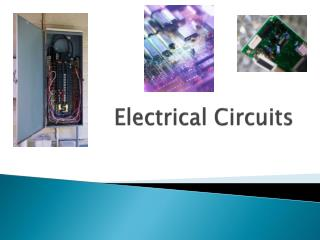DownloadDownload PresentationElectrical Circuits

Electrical Circuits

Télécharger la présentationElectrical Circuits

- - - - - - - - - - - - - - - - - - - - - - - - - - - E N D - - - - - - - - - - - - - - - - - - - - - - - - - - -
Presentation Transcript

1. Electrical Circuits

2. Circuits • Electricity flowing through a substance is called an electric current. • A circuit is a path through which electricity flows from a negative terminal to a positive terminal. • The standard unit for the measure of current is the ampere, which is often abbreviated “amp.” • The amount of force per electron flowing through a substance is called voltage.

3. Requirements for Electricity to Flow Through a Circuit • A voltage source must be present. • The voltage source must be connected to a material through which the electric current can flow. • A circuit must be complete for the electrons to flow. If the path is broken, the current stops flowing.

4. Schematic Symbols • A drawing of an electric circuit is called a schematic. • Symbols • Resistor • Battery Source • Switch

5. More about Schematics • The opposition to the flow of electrons is called resistance. The resistor may also be called the load and is the part of the circuit that converts the electrical energy into another form. (Such as light bulbs). Resistance is measured in ohms. An ohm is equivalent to one volt per ampere. • The source of current is also the voltage. • The switch opens and closes the circuit. The circuit must be closed in order for electricity to flow.

6. Series and Parallel Circuits • Series circuit - has only one path through which the electricity can flow. • Parallel circuit - has multiple paths through which the electricity can flow.

7. Switches • Switches are used to control circuits. • Switches connect or disconnect all or part of a circuit. • Switches come in many forms. • SPST • SPDT • DPST • DPDT

8. More about Types of Switches • The first and simplest is a Single Pole Single Throw (SPST). This is a basic on/off switch. • A Single Pole Double Throw (SPDT) is basically two Single Pole Single Throw switches tied together so that they function together.

9. More Types of Switches • Another type of switch is a Double Pole Single Throw (DPST). This switch is used for dual switch control of a single circuit, such as two switches to control one light in your house. • A fourth type of switch is a Double Pole Double Throw (DPDT). This is basically two Double Pole Single Throw switches tied together so that they function together.

10. Integrated Circuits • Transistors are small electronic devices that contain a semiconductor and have at least three electrical contacts. They were invented in 1947. • An integrated circuit is a complete circuit on a piece of semiconductor material. Integrated circuits were invented in 1958 and led to even smaller, less-expensive electronics and eventually to the development of the microprocessor.